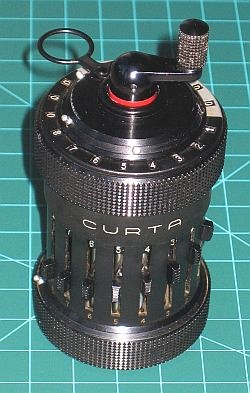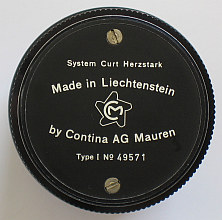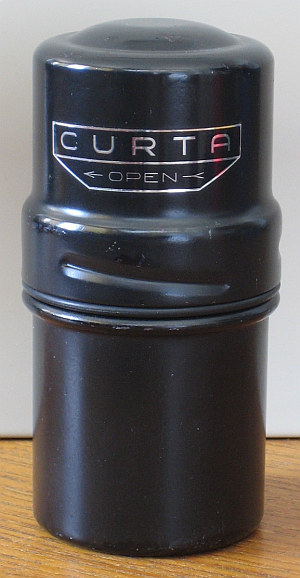previous <<==>> next

CURTA-1``` T E C H N I C A L D A T A of the Mechanical Calculator CURTA-1 ******************************************************************* * CURTA-1 & CURTA-2 , the world's s m a l l e s t * * full-functional m e c h a n i c a l calculators ... * * * * Be sure to look at ... * * * * ===>>> www.CURTA.de , Jan Meyer's exciting Site ... * * and * * ===>>> SCIENTIFIC AMERICAN, Jan.2004, p.92-99, Cliff Stoll's * * "The Curious History of the First Pocket Calculator" * ******************************************************************* Serial Number: 49571 Dimensions: Diameter = 2_1/16" / 53 mm Height = 4_1/8 " / 105 mm Weight: 8 oz / 230 g Mechanics: System Curt Herzstark Stepped Drum / Staffelwalze Functions: Add, Subtract, Multiply, Divide Registers: Input = 8 Decimals Counter = 6 Decimals Arithmetic = 11 Decimals Manufacturer: CONTINA AG Vaduz / Liechtenstein 1950 P A R T S of the C U R T A - 1 ************************************************* Looks different, but works like a big Calculator! <<<<< <<<<< for Subtraction, Division <<<<<---------- pulled up RED MARK shown <<<<< C R A N K Z (Turns CLOCKWISE only!) <<<<<---------- pushed down for Addition, Multiplication OOOOOOOOOOOOOOO O O O CLEARING LEVER can be folded over OOOOOOOOOOOOOOO when stored into container +- Decimal MARKERS are shiftable -----------+ | ARITHMETIC UNIT with 11 black dials | | COUNTER UNIT with 6 white dials | +->> C A R R I A G E turnable when lifted <<-+ with Position Numbers 11 ... 1 +->> B O D Y Front Side <<-+ | INPUT DIALS 8 ... 1 | | | | 0 = up | | INPUT SLIDERS Z in 8 columns | | 9 = down | | | | SLOT NUMBERS 8 ... 1 | +--- Decimal MARKERS are shiftable -----------+ +-<< B O D Y Rear Side (not shown) >>-+ | up = normal | | REVERSING LEVER Z for counting | +---------------- down = reverse -----------+ H O W T O U S E the CURTA-1 ********************************* BEFORE STARTING A TASK: ----------------------- All INPUT SLIDERS and the REVERSING LEVER in upper position. CARRIAGE in position 1. COUNTER and ARITHMETIC UNIT cleared. CRANK in down position. ADDITION & SUBTRACTION: ----------------------- Example: 123 + 45 - 6 = 162 ADD: Enter the first number (123) in the far right columns with the input sliders. Turn the crank to transfer the number into arithmetic unit. The counting unit displays the figure 1. Enter the second number (45). Make a turn with the crank to add the number. The arithmetic unit displays the intermediate sum (168) and the counting unit displays the figure 2. SUBTRACT: Enter the third number (6). Pull up the crank (red mark shown) and make a turn. The arithmetic unit displays the result (162) and the counting unit is decreased by 1. REMARK: NEGATIVE RESULTS are displayed in the arithmetic unit as the COMPLEMENT of the next higher 10, 100, 1000, ... Example: -12 = 99...9988 MULTIPLICATION: --------------- Example: 123 x 45 = 5535 Enter the multiplicand (123) in the far right input columns. The multiplicator (45) has two digits, so the arithmetic unit (in the carriage) is moved to position 2. Repeat making turns with the crank, until the first figure of the multiplicator (4) will appear in the 2nd position of the counter unit. Move the arithmetic unit to position 1. Repeat making turns with the crank, until the second figure of the multiplicator (5) appears in the 1st position of the counter unit. The multiplicand (123) stays in the input dials, the multiplicator (45) in the counter unit and the result (5535) is in the arithmetic unit. DIVISION: --------- Example: 22 : 7 = 3.14285 Remainder 5 Division requires 3 steps: (A) To Set the Dividend into Arithmetic Unit: ----------------------------------------- For the maximum number of decimals, set the arithmetic unit to the far left position 6. Enter the dividend (22) in the far right input columns. Make a turn with the crank to transfer into arithmetic unit. (B) To Set the Divisor into Input Unit: ----------------------------------- Clear the counter unit. Shift the reversing lever down. Enter the divisor (7) below the dividend (22). Pull up the crank (red mark shown). (C) To Divide: ---------- Make (subtract) turns with the crank until the arithmetic unit shows an "underflow". Push down the crank, make one (add) turn and pull up the crank again. Move the carriage to the next right position. Repeat this procedure until the required numbers of decimals are calculated... The result (3.14285) is in the counter unit, and the remainder (5) is in the arithmetic unit. The divisor (7) stays in the input dials, therefore an additional decimal can be estimated... ( 7, cause 7 x 7 = 49 ). Have a look at "Calculating Trickies" ...impressum: ******************************************************************* © C.HAMANN http://public.BHT-Berlin.de/hamann 09/11/09 ```